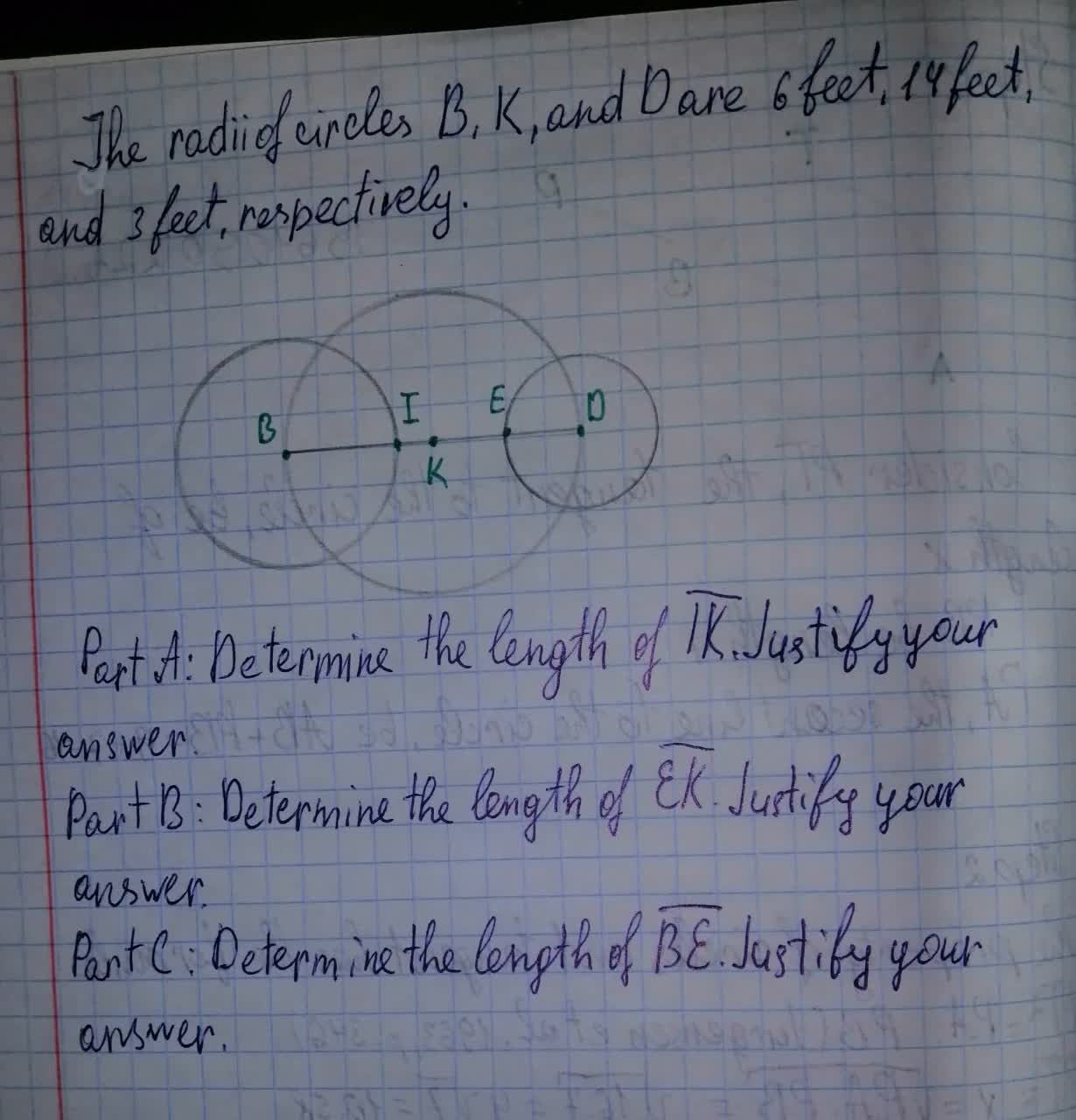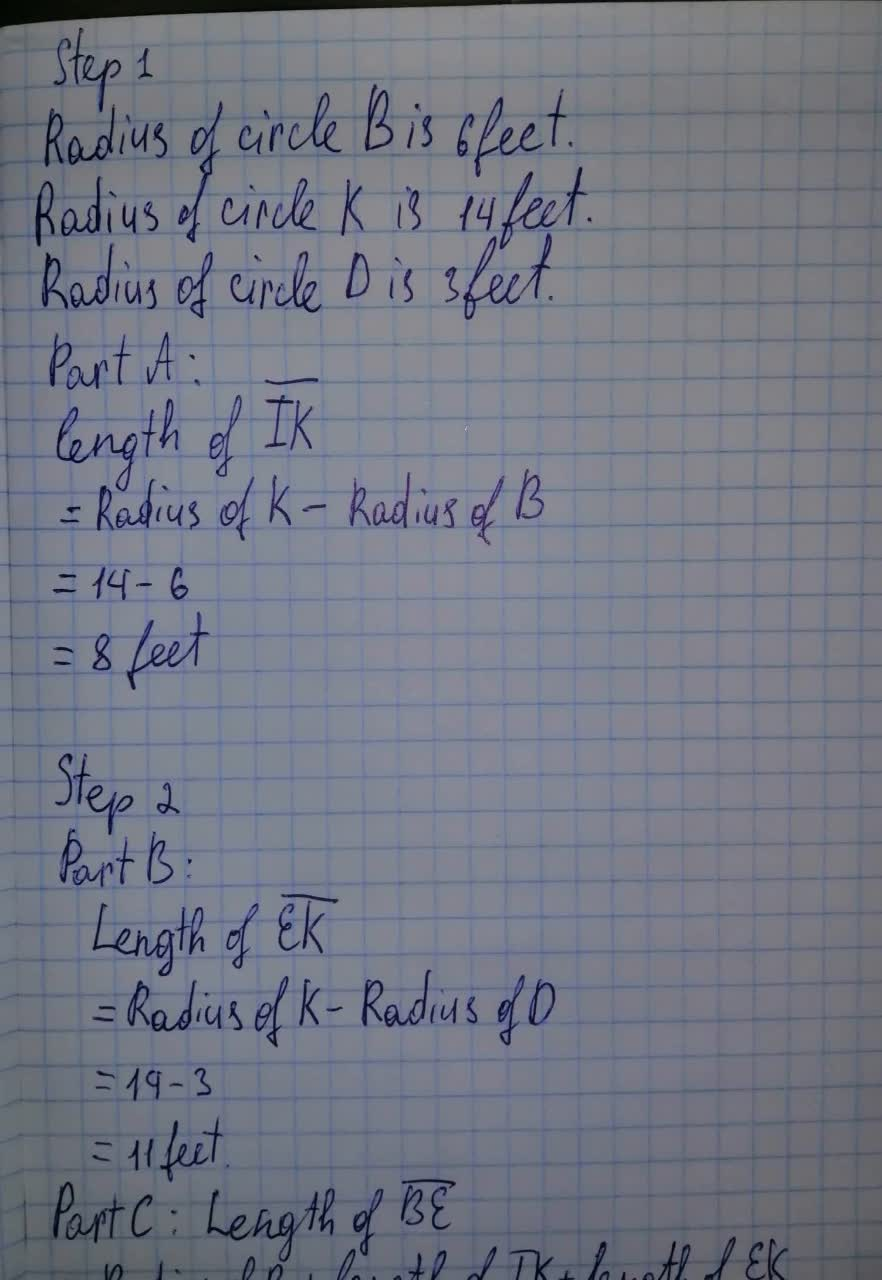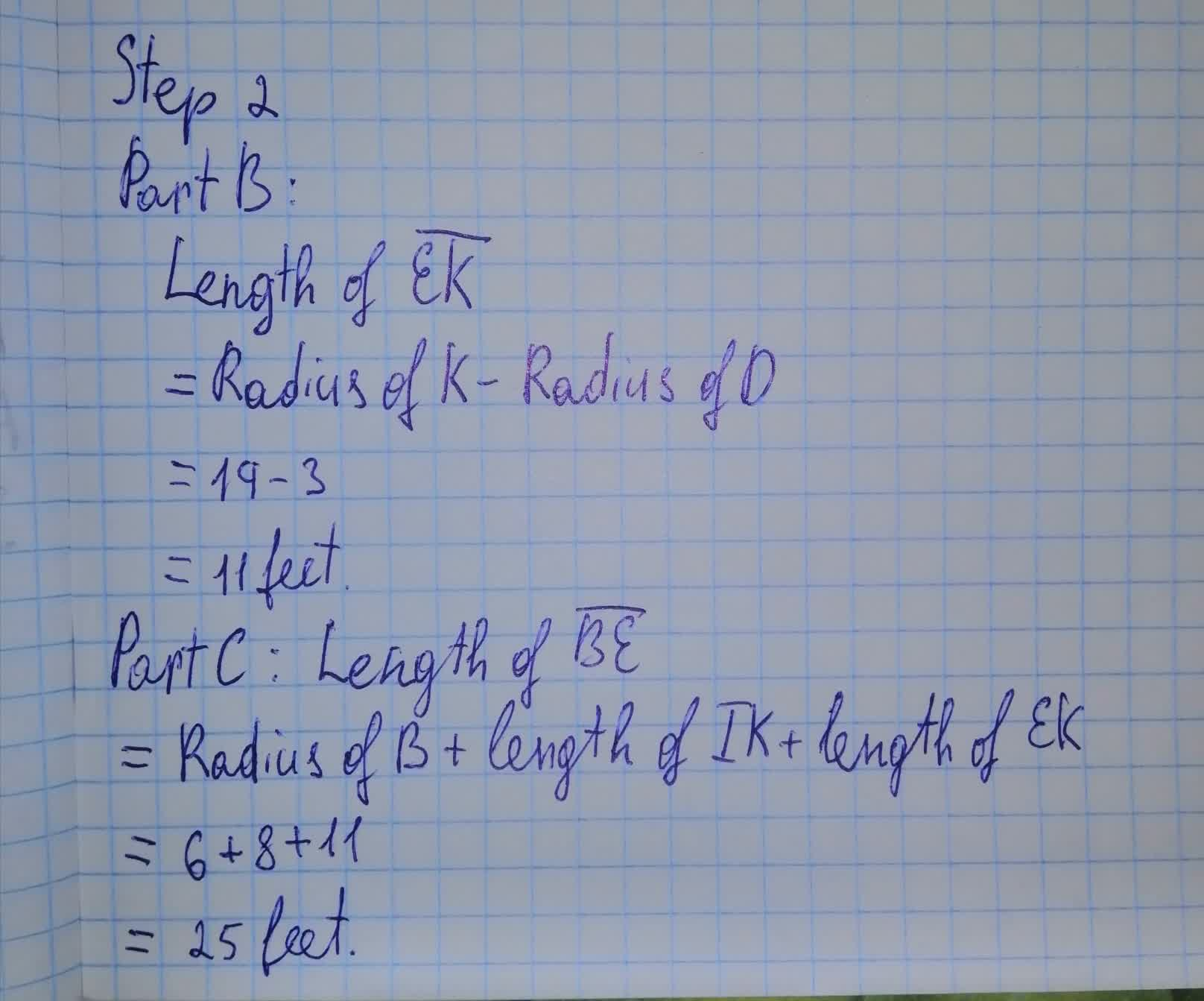Question# The radii of circles B,K, and D are 6 feet ,14 feet ,and 3 feet , respectively.Part A: Determine the length of \overline{IK}. Justify your answer.

Circles
ANSWEREDThe radii of circles B,K, and D are 6 feet ,14 feet ,and 3 feet , respectively
Part A: Determine the length of $$\displaystyle\overline{{{I}{K}}}$$. Justify your answer.
Part B: Determine the length of $$\displaystyle\overline{{{E}{K}}}$$. Justify your answer.
Part C: Determine the length of $$\displaystyle\overline{{{B}{E}}}$$. Justify your answer.